Trial ends in

# Determination of the Gas-phase Acidities of Oligopeptides

doi: 10.3791/4348 Published: June 24, 2013

### Summary

The determination of the gas-phase acidities of cysteine-containing oligopeptides is described. The experiments are performed using a triple quadrupole mass spectrometer. The relative acidities of the peptides are measured using collision-induced dissociation experiments, and the quantitative acidities are determined using the extended Cooks kinetic method.

### Abstract

Amino acid residues located at different positions in folded proteins often exhibit different degrees of acidities. For example, a cysteine residue located at or near the N-terminus of a helix is often more acidic than that at or near the C-terminus 1-6. Although extensive experimental studies on the acid-base properties of peptides have been carried out in the condensed phase, in particular in aqueous solutions 6-8, the results are often complicated by solvent effects 7. In fact, most of the active sites in proteins are located near the interior region where solvent effects have been minimized 9,10. In order to understand intrinsic acid-base properties of peptides and proteins, it is important to perform the studies in a solvent-free environment.

We present a method to measure the acidities of oligopeptides in the gas-phase. We use a cysteine-containing oligopeptide, Ala3CysNH2 (A3CH), as the model compound. The measurements are based on the well-established extended Cooks kinetic method (Figure 1) 11-16. The experiments are carried out using a triple-quadrupole mass spectrometer interfaced with an electrospray ionization (ESI) ion source (Figure 2). For each peptide sample, several reference acids are selected. The reference acids are structurally similar organic compounds with known gas-phase acidities. A solution of the mixture of the peptide and a reference acid is introduced into the mass spectrometer, and a gas-phase proton-bound anionic cluster of peptide-reference acid is formed. The proton-bound cluster is mass isolated and subsequently fragmented via collision-induced dissociation (CID) experiments. The resulting fragment ion abundances are analyzed using a relationship between the acidities and the cluster ion dissociation kinetics. The gas-phase acidity of the peptide is then obtained by linear regression of the thermo-kinetic plots 17,18.

The method can be applied to a variety of molecular systems, including organic compounds, amino acids and their derivatives, oligonucleotides, and oligopeptides. By comparing the gas-phase acidities measured experimentally with those values calculated for different conformers, conformational effects on the acidities can be evaluated.

### Introduction

The acidities of amino acid residues are among the most important thermochemical properties that influence the structures, the reactivity, and the folding-unfolding processes of proteins 9,19. Individual amino acid residues often show different effective acidities depending on their locations in proteins. In particular, the residues located at the active sites often exhibit significantly perturbed acidities. One such example is the cysteine residue residing in the active sites of the thioredoxin super-family of enzymes 20,21. The active site cysteine is unusually acidic compared to those in unfolded proteins 3-5. It has been suggested that the helical conformation may have a significant contribution to the unusual acidity. There are extensive experimental studies on the acid-base properties of peptides carried out in solutions, especially in aqueous solutions 2,6-8. The results were often complicated by solvent effects 7. In fact, most of the active sites in proteins are located near the interior region where solvent effects are minimized 9,10.

In order to understand intrinsic acid-base properties of peptides and proteins, it is important to carry out the studies in a solvent-free environment. Here we introduce a mass spectrometry-based method for the determination of the gas-phase acidity. The approach is referred to as the extended Cooks kinetic method. This method has been successfully applied to a wide range of chemical systems for the determination of various thermochemical properties, such as the gas-phase acidity, the proton affinity, the metal ion affinity, the electron affinity, and the ionization energy 11-15,22-26. We have applied this method to determine the gas-phase acidities of a series of oligo cysteine-polyalanine and cysteine-polyglycine peptides 17,18,27. These studies show that the N-terminal cysteine peptides are significantly more acidic than the corresponding C-terminal ones. The high acidities of the former are likely due to the helical conformational effects in which the thiolate anion is strongly stabilized by the interaction with the helix macro-dipole. Because of the non-volatile and thermally labile nature of peptides, the kinetic method is the most practical approach available at present to produce reasonably accurate acid-base thermochemical quantities of peptides 28.

The general scheme and the equation associated with the kinetic method are shown in Figure 1. The determination of the gas-phase acidity of a peptide (AH) starts with the formation of a series of proton-bound cluster anions, [A•H•Ai]¯ (or [A¯•H+•Ai¯]¯), in the ion source region of the mass spectrometer, where A¯ and Ai¯ are the deprotonated forms of the peptide and the reference acids, respectively. The reference acids are organic compounds with known gas-phase acidities. The reference acids should have structures similar to each other (but not necessarily similar to that of the peptide). The similarity of the structures between reference acids ensures the similarity of the entropies of deprotonation among them. The proton-bound cluster anions are mass selected and collisionally activated and subsequently dissociated using collision-induced dissociation (CID) experiments to yield the corresponding monomeric anions, A¯ and Ai¯, with rate constants of k and ki, respectively, shown in Figure 1a. If secondary fragmentations are negligible, the abundance ratio of the CID fragment ions, [A¯]/[Ai¯], represents an approximate measure of the ratio of the rate constants, k/ki. Under the assumption that there are no reverse activation barriers for both dissociation channels, the CID product ion branching ratios, ln[A¯]/[Ai¯], will be linearly correlated to the gas-phase acidity of the peptide (ΔacidH) and those of the reference acids (ΔacidHi), as shown in Figure 1b. In this equation, ΔacidHavg is the average gas-phase acidity of the reference acids, Δ(ΔS) is the entropy term (which can be assumed constant if the reference acids are structurally similar to each other), R is the universal gas constant, and Teff is the effective temperature of the system. The effective temperature is an empirical parameter that depends on several experimental variables, including the collision energy.

The value of the gas-phase acidity is determined by constructing two sets of thermo-kinetic plots. The first set is obtained by plotting ln([A¯]/[Ai¯]) against ΔacidHi - ΔacidHavg, as shown in Figure 4a. Linear regression will yield a set of straight lines with the slopes of X = 1/RTeff and intercepts of Y = - [ΔacidH - ΔacidHavg]/RTeff - Δ(ΔS)/R. The second set of plots is obtained by plotting the resulting intercepts (Y) from the first set against the corresponding slopes (X), as shown in Figure 4b. Linear regression produces a new line with a slope of ΔacidH - ΔacidHavg and an intercept of Δ(ΔS)/R. The value of ΔacidH is then obtained from the slope and the entropy term, Δ(ΔS), is obtained from the intercept.

The experiments are performed using a triple quadrupole mass spectrometer interfaced to an electrospray ionization (ESI) ion source. A schematic diagram of the mass spectrometer is shown in Figure 2. The CID experiments are performed by mass selecting the proton-bound cluster anions with the first quadrupole unit and allowing them to undergo collisions with argon atoms leaked into the collision chamber which is held at a pressure of around 0.5 mTorr. The dissociation product ions are mass analyzed with the third quadrupole unit. The CID spectra are recorded at several collision energies with the m/z range wide enough to cover all possible secondary fragments. The CID product ion intensities are measured by setting the instrument in the selected reaction monitoring (SRM) mode in which the scan is focused on selected product ions. The CID experiments are performed at four different collision energies, corresponding to center-of-mass energies (Ecm) of 1.0, 1.5, 2.0, and 2.5 eV, respectively. The center-of-mass energy is calculated using the equation: Ecm = Elab [m/(M+m)], where Elab is the collision energy in the laboratory frame, m is the mass of argon, and M is the mass of the proton-bound cluster ion.

In this article, we use the oligopeptide Ala3CysNH2 (A3CH) as the model compound. The C-terminus is amidated and the thiol group (SH) of the cysteine residue will be the acidic site. The selection of the suitable reference acids is crucial for the successful measurement of the gas-phase acidity. The ideal reference acids are structurally similar (to each other) organic compounds with well-established gas-phase acidity values. The reference acids should have acidity values close to that of the peptides. For the peptide A3CH, six halogenated carboxylic acids are chosen as the reference acids. The six reference acids are chloroacetic acid (MCAH), bromoacetic acid (MBAH), difluoroacetic acid (DFAH), dichloroacetic acid (DCAH), dibromoacetic acid (DBAH), and trifluoroacetic (TFAH). Two of them, DFAH and MBAH, will be used to illustrate the protocol.

### Protocol

1. Preparation of the Sample Solutions

1. First prepare the stock solutions of the peptide and the six reference acids using a mixed solvent of methanol and water in a 1:1 volume ratio. The stock solutions should have a concentration of about 10-3 M.
2. Weigh out 1 mg of the solid peptide sample, A3CH, in a 1.5 ml Eppendorf tube and add 1.0 ml of mixed solvent of methanol and water, and mix using a vortex.
3. Weigh out 1 mg of difluoroacetic acid (DFAH) and add 1.0 ml of the mixed solvent of methanol and water, and mix using a vortex.
4. Use the same procedure to make the stock solutions for the other five reference acids chloroacetic acid (MCAH), bromoacetic acid (MBAH), dichloroacetic acid (DCAH), dibromoacetic acid (DBAH), and trifluoroacetic acid (TFAH).
5. Draw about 50 μl of the peptide stock solution into a 1.5 ml Eppendorf tube, and draw about 50 μl of the stock solution of DFAH into the same Eppendorf tube. Dilute the mixed solution with 900 μl of the mixed solvent of methanol and water to achieve a final concentration of about 10-4 M. This diluted solution will be used as the sample solution for the mass spectrometry measurements. The actual ratio of the peptide to the reference acid as well as the final concentration of the sample solution will be adjusted based on the ion signal abundances observed in the mass spectrometer.
6. Use the same procedure to prepare the sample solutions of the peptide with the other five reference acids.

2. Mass Spectrometry Measurement 1: Proton-bound Cluster Ion Formation

1. The first step of the mass spectrometry measurement is to generate stable proton-bound cluster ions of the peptide with a reference acid.
2. Set the instrument in the negative ion MS mode with the ESI needle voltage at -4.5 kV, the capillary voltage at about -35 V, and the drying gas temperature maintained at 150 °C. Set the Q1 peak width and the Q3 peak width both to the calibrated scale (The peak width is an instrument parameter that can be used to adjust the resolution of the peaks. The "calibrated scale" setting allows to display narrow peaks for better peak separation). The capillary voltage and the drying gas temperature can be adjusted to enhance the observed ion abundances.
3. Infuse about 0.5 ml of the sample solution of peptide-DFAH into a 1 ml Hamilton syringe and connect the syringe to the ESI needle inlet using PEEK tubing. Then place the syringe onto the syringe pump. Turn on the syringe pump to infuse the sample solution into the ESI needle with the flow rate at 10 μl/min.
4. Turn on the ESI needle voltage to activate the ESI process. Turn on the detector. A mass spectrum display should be observed in the profile mode. If the display is in the centroid mode, switch to the profile mode. Watch the proton-bound cluster ion formation by monitoring the peak at m/z 428. The signal abundance of the cluster ion can be adjusted by fine tuning the instrument. One important parameter is the capillary voltage. One can manually change the capillary voltage (typically in the range of -20 to -50 V) to maximize the abundance of the peak at m/z 428.

3. Mass Spectrometry Measurement 2: The CID Bracketing Experiments

1. The next step is to perform the CID bracketing experiment.
2. Once the abundance of the cluster ion reaches the desired value (around 100 mV), switch the instrument to the MS/MS mode. In this mode, Q1 functions as a mass filter to isolate the cluster ion, Q2 functions as the collision cell, and Q3 functions as the mass analyzer.
3. Set the collision gas (argon, in this case) pressure at 0.5 mTorr and the collision energy at 17 eV. Three peaks should be observed in the mass spectrum display window. The peak at m/z 428 corresponds to the cluster ion, [DFA•H•A3CS]¯. The two peaks at m/z 332 and m/z 95 correspond to the deprotonated peptide (A3CS¯) and the deprotonated difluoroacetic acid (DFA¯), respectively. The minor peak at m/z 298 is a secondary fragment from the deprotonated peptide. Acquire a CID spectrum for 2 min, Figure 3a.
4. Perform similar CID experiments and acquire a CID spectrum for the sample solution of the peptide with bromoacetic acid (MBAH), Figure 3b.
5. Perform similar CID experiments and acquire the CID spectra for the sample solutions of the peptide with all other reference acids. The resulting CID spectra will be qualitatively similar to Figures 3a and 3b, but the m/z values and the relative peak heights will be different.

4. Mass Spectrometry Measurement 3: The Kinetic Method

1. The last step is to acquire the SRM spectra.
2. Switch the spectrum display to centroid and set the instrument to the selected reaction monitoring (SRM) mode. Keep m/z 428 as the isolated ion by the first quadrupole (Q1), and fill in four masses (mass-to-charge ratios) to be monitored by the third quadrupole (Q3). The four masses are m/z 428 (the cluster ion), m/z 332 (the peptide ion), m/z 298 (the fragment of the peptide ion), and m/z 95 (the DFA¯ ion). Keep the collision gas pressure at 0.5 mTorr.
3. Set the collision energy to 11.7 eV and acquire the spectra for 5 min.
4. Change the collision energy to 17.6 eV and acquire the spectra for 5 min.
5. Change the collision energy to 23.4 eV, and 29.3 eV, and acquire the spectra at both collision energies for 5 min.
6. Perform similar measurements for the peptide with all other reference acids.

5. Data Analysis

1. Copy the values of the ion intensities from all the SRM spectra onto an Excel worksheet.
2. Calculate the CID product ion branching ratios, ln([A¯]/[Ai¯]), measured for all six proton-bound clusters at all four collision energies. Sample values are shown in Table 1.
3. Plot the values of ln([A¯]/[Ai¯]) against the values of ΔacidHi - ΔacidHavg. This will give four plots corresponding to the data at four collision energies, Figure 4a.
4. Extract the values of the slopes and the intercepts by linear regression of the four plots. In this case, the slopes are positive values and the intercepts are negative values. Give the symbol "X" to the slopes and the symbol "Y" to the intercepts. The results are shown in Table 2. Multiply the values of Y by -1 and use the symbol Y' to represent the positive values (this allows the y-axis to display positive values). Note, this conversion is optional as long as the corresponding values are used to make the plot for the next step.
5. Plot the values of Y' against the values of X, Figure 4b. Linear regression of the plot yields a slope of 1.706 and an intercept of -0.536. The slope corresponds to ΔacidH - ΔacidHavg. The value of ΔacidHavg is known to be 330.5 kcal/mol, which is determined by the set of selected reference acids. The value of the gas-phase acidity of the peptide is then obtained from the slope: ΔacidH(A3CH) = 332.2 kcal/mol.

### Representative Results

1. The CID bracketing experiments provide information on the relative acidities of the peptide compared to the selected reference acids. Two representative CID spectra of the peptide (A3CH) with two reference acids, DFAH and MBAH, are shown in Figure 3. In Figure 3a the ion abundance (peak height) of the peptide ion is weaker than that of DFA¯, and in Figure 3b, the ion abundance of the peptide ion is stronger than that of MBA¯. The two spectra suggest that the gas-phase acidity of the peptide is in the range between the acidities of these two reference acids.
2. The quantitative value of the gas-phase acidity of the peptide is determined from the quantitative CID experiments. The thermo-kinetic plots for the dissociation of the proton-bound clusters of the peptide with the six reference acids are shown in Figure 4. Linear regression of the plots according to the thermo-kinetic relationship between the gas-phase acidity and the CID product ion branching ratio (Figure 1b) gives the value of the gas-phase acidity of the peptide A3CH, which is 332.2 kcal/mol. Sample values of the slopes and intercepts are shown in Tables 1 and 2.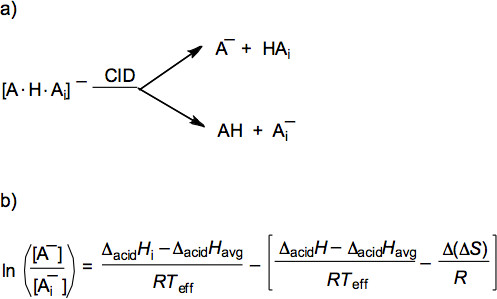Figure 1. The overall scheme of the extended Cooks kinetic method. a) The scheme of the proton-bound cluster ion dissociation. b) The thermo-kinetic relationship between the gas-phase acidities and the CID product ion branching ratio. In this equation, ΔacidHi is the gas-phase acidity value of the individual reference acids, ΔacidHavg is the average gas-phase acidity of the reference acids, ΔacidH is the gas-phase acidity for the peptide, Δ(ΔS) is the entropy term, R is the universal gas constant, and Teff is the effective temperature of the system.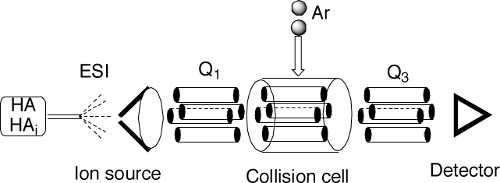Figure 2. A schematic drawing of a triple-quadrupole mass spectrometer. ESI is the electrospray ionization ion source. Q1 and Q3 represent the first and third quadrupole units, respectively. Upon performing the CID experiment, the proton-bound cluster ions are mass selected by Q1, and are guided into the collision cell to collide with the argon (Ar) atoms leaked into the collision cell, and the resulting fragment ions are analyzed by Q3.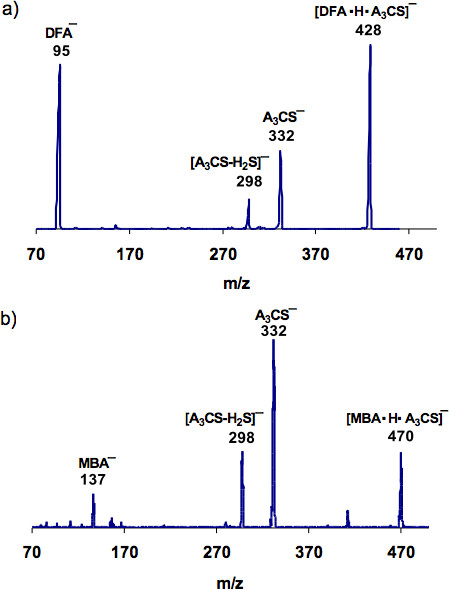Figure 3. The CID spectra for the proton-bound cluster ions of the peptide with two reference acids, a) [DFA•H•A3C]¯ and b) [MBA•H•A3C]¯. The spectra are plotted as the relative ion abundance against the m/z value.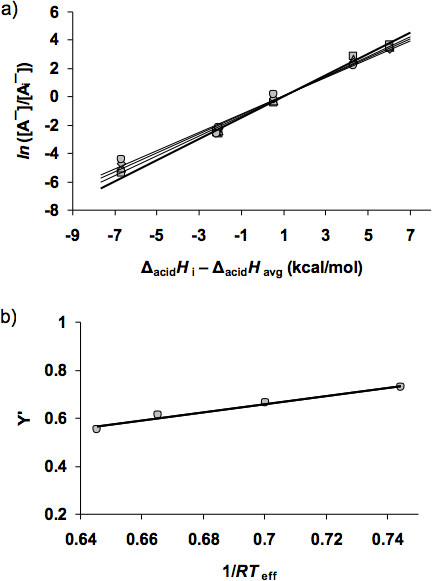Figure 4. The thermo-kinetic plots for the peptide with six reference acids collected at four collision energies. a) The plot of Y = ln([A¯]/[Ai¯]) against X = ΔacidHi - ΔacidHavg. b) The plot of Y' = [ΔacidH - ΔacidHavg]/RTeff - Δ(ΔS)/R against X = 1/RTeff.

 HAi 11.7 eV 17.6 eV 23.4 eV 29.3 eV MCAH 3.68 3.50 3.39 3.45 MBAH 2.83 2.65 2.45 2.24 DFAH -0.442 -0.268 -0.0921 0.167 DCAH -2.60 -2.41 -2.22 -2.13 DBAH -2.43 -2.44 -2.49 -2.60 TFAH -5.41 -5.02 -4.71 -4.44

Table 1. Values of CID product ion branching ratios, ln([A¯]/[Ai¯]), for the cluster ions of the peptide with DFAH and MBAH.

 Ecolli, eV X 1/RTeff Y- [(ΔacidH - ΔacidHavg)/RTeff - Δ(ΔS)/R] 11.7 0.744 -0.728 17.6 0.700 -0.665 23.4 0.665 -0.611 29.3 0.645 -0.553

Table 2. Values of the slopes (X) and the intercepts (Y) resulting from linear regression of the first set of thermo-kinetic plots.

### Discussion

The successful measurement of the gas-phase acidity of a peptide largely relies on the selection of suitable reference acids. The ideal reference acids are structurally similar organic compounds with well-established gas-phase acidity values. The reference acids should have similar structures to each other. This will ensure a similar entropy of deprotonation for each of the reference acids in the set. The reference acids should have acidity values close to those of the peptides. For shorter cysteine-containing oligopeptides with amidated C-termini, the halogenated carboxylic acids are suitable reference acids. A crucial step toward successful CID experiments is the formation of the stable proton-bound cluster ions with high abundance. The stability and the abundance can be largely improved by adjusting the ratio of the peptide to the reference acid, the concentration of the sample solution, and the instrumental conditions (such as the needle voltage, the drying gas temperature, and the capillary voltage). One crucial instrumental condition is the capillary voltage (or cone voltage for some other types of instruments). One caution is to avoid using sample solutions that are too concentrated.

The method described is not limited to oligopeptides. The method can be applied to a variety of molecular systems, including polar organic compounds, amino acids and their derivatives, organometallic compounds, oligonucleotides, and peptide-mimicking polymers. In addition to using the triple-quadrupole mass spectrometer, the experiment can also be carried out using ion traps and Q-TOF mass spectrometers.

The peptide used in this experiment was synthesized in our laboratory using the standard method of solid phase peptide synthesis 29-31. The Rink amide resin was used as the solid support to yield the amide C-terminus. The advantage of the Cooks kinetic method is that minor impurities in the peptide sample do not influence the acidity measurements, as long as the impurities do not have the same masses as the peptides or the reference acids.

The experimental measurements can be coupled with computational studies to examine conformational effects on the acidities. Computational studies provide predictions of the conformations of the peptides that match their calculated acidities. By comparing the acidities measured experimentally with the values calculated, the conformations of the peptides can be evaluated.

### Disclosures

Nothing to disclose.

### Acknowledgments

The work was supported by the National Science Foundation (CHE-0749737). The instrument usage was provided by the Mass Spectrometry Facility at the University of the Pacific.

### Materials

 Name Company Catalog Number Comments Mass Spectrometer Varian 1200 L and 320 L Chloroacetic acid Sigma-Aldrich 402923 Bromoacetic acid Sigma-Aldrich B56307 Difluoroacetic acid Sigma-Aldrich 142859 Dichloroacetic acid Sigma-Aldrich D54702 Dibromoacetic acid Sigma-Aldrich 242357 Trifluoroacetic acid Sigma-Aldrich T6508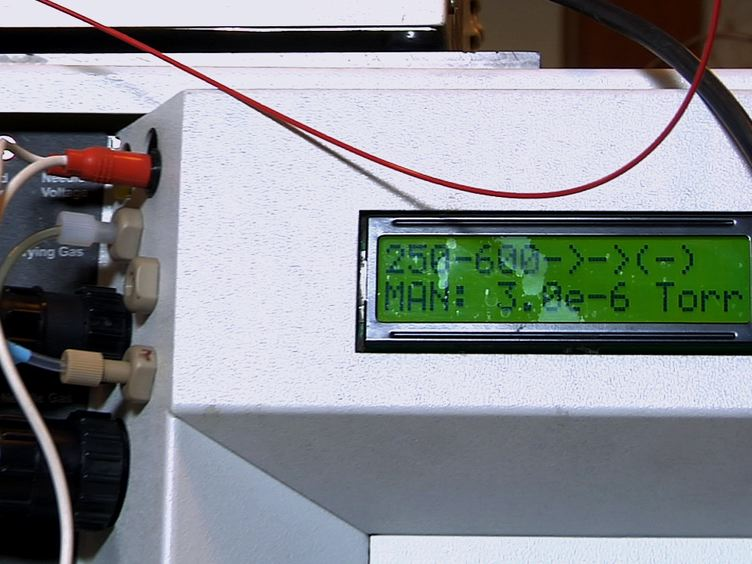Play Video
DOIX